Function Repository Resource:

# KroneckerPoint

Get a quasirandom point based on Kronecker sequences

Contributed by: Ed Pegg Jr
 ResourceFunction["KroneckerPoint"][n] return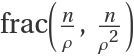, where ρ is the plastic constant. ResourceFunction["KroneckerPoint"][dim,n] return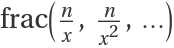where x is a real solution of xdim+1=x+1.

## Details

The golden ratio is the real solution for ϕ2=ϕ+1, ϕ≈1.61803398874989.
The plastic ratio is the real solution for ρ3=ρ+1, ρ≈1.32471795724475.
The plastic sequence is a 2D quasirandom sequence (also called a low-discrepancy sequence).

## Examples

### Basic Examples (3)

Generate the first point:

 In:=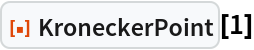Out=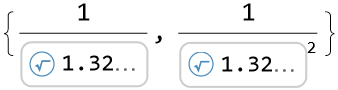Numeric approximation of the first three points:

 In:=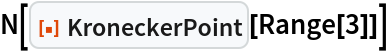Out=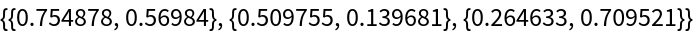The first three points with ρ (rho) as the plastic constant:

 In:=Out=### Scope (3)

 In:=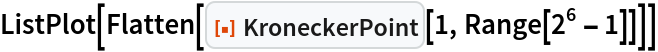Out=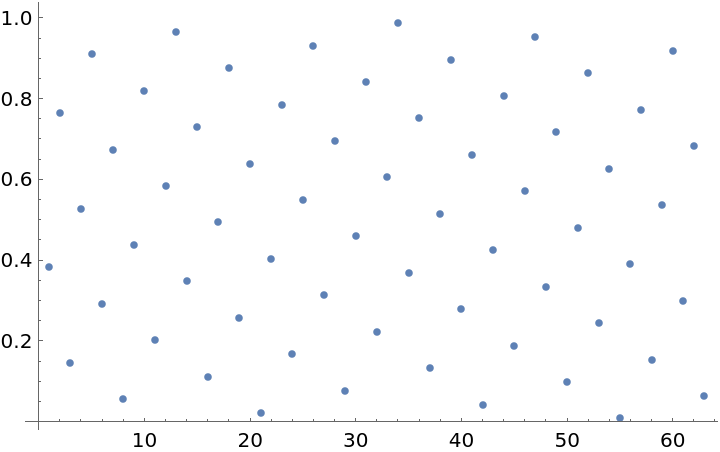Show the structure of the plastic quasirandom sequence:

 In:=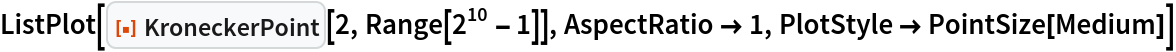Out=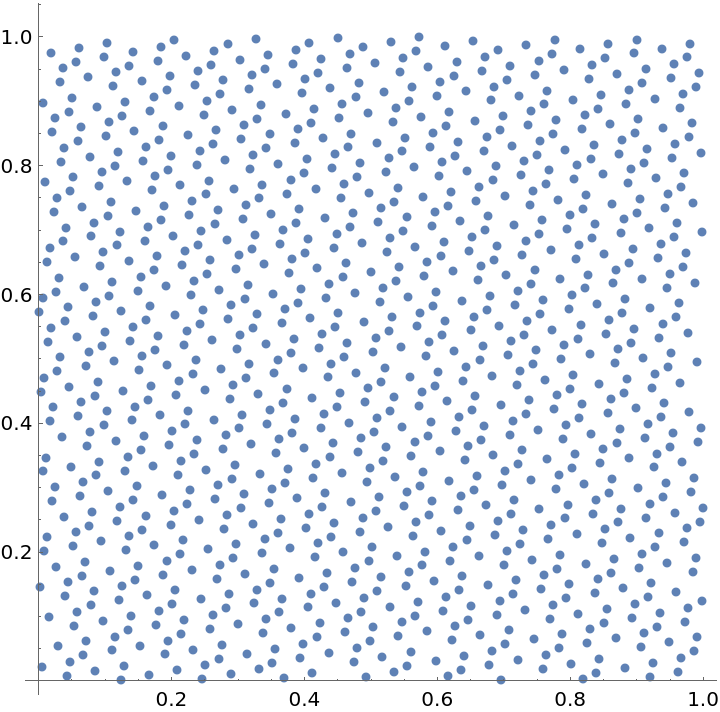The first 500 points as Voronoi cells:

 In:=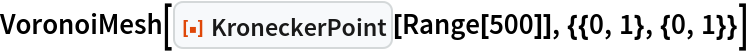Out=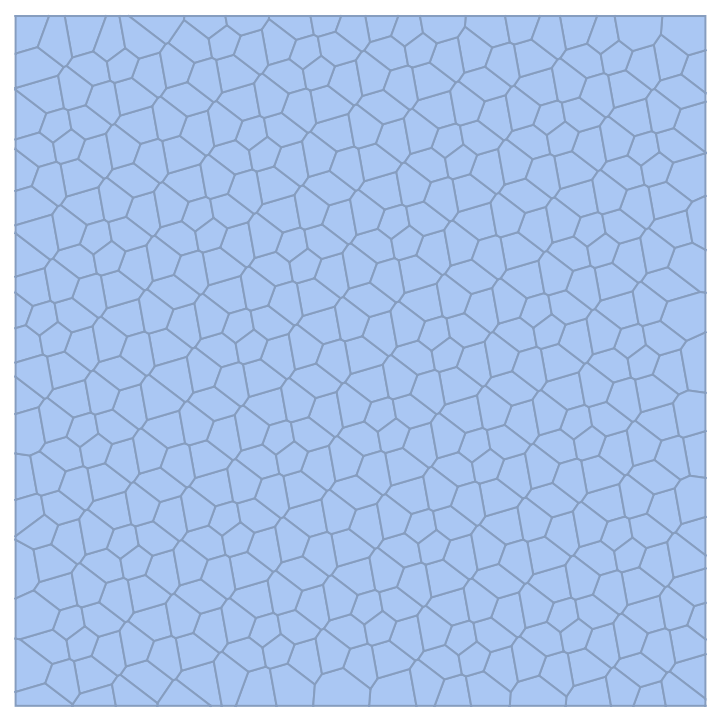Show the dimension 3 points:

 In:=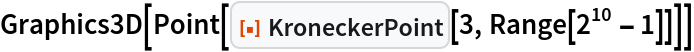Out=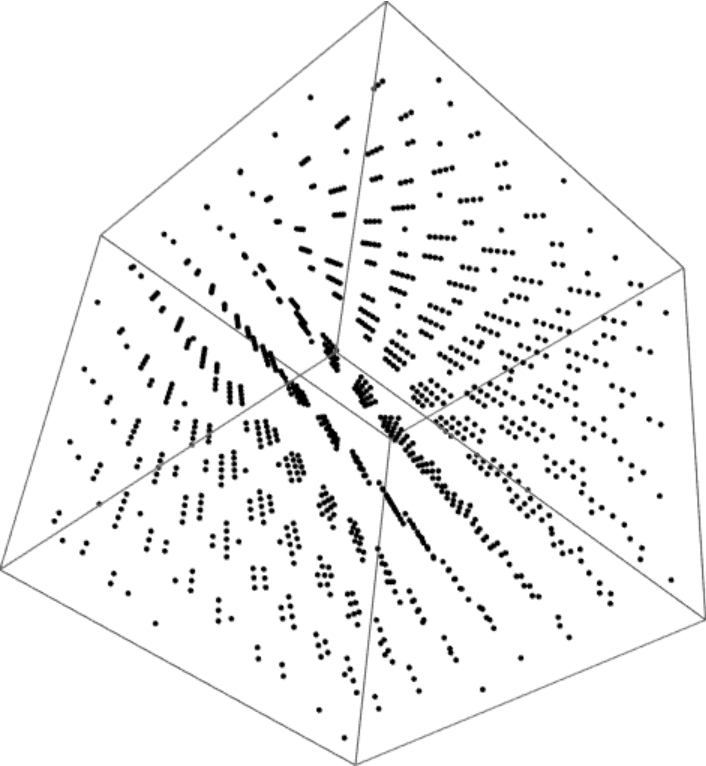### Neat Examples (1)

The plastic sequence can be folded into a triangle and still be a low-discrepancy sequence:

 In:=Out=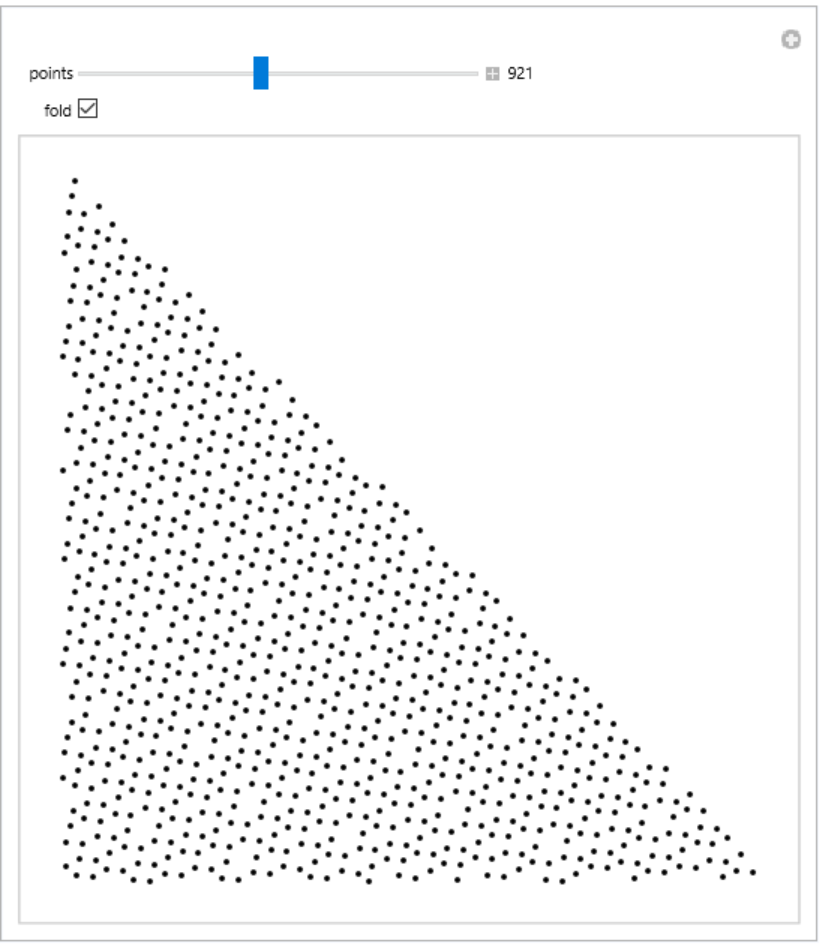## Version History

• 1.0.0 – 02 December 2022